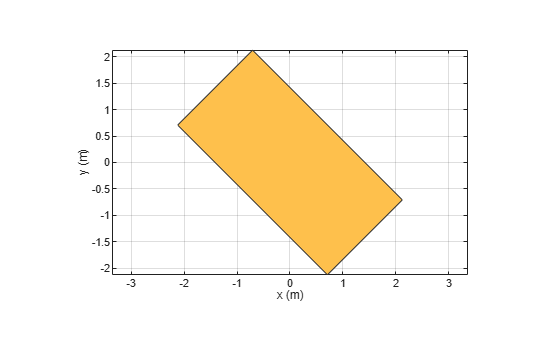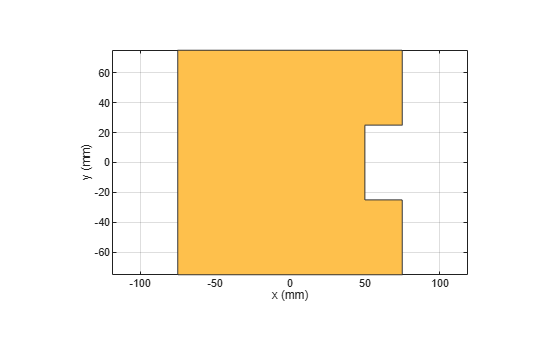antenna.Rectangle

Create rectangle centered at origin on X-Y plane

Description

Use the antenna.Rectangle object to create a rectangle centered at the origin and on the X-Y plane. You can use antenna.Polygon to create single-layer or multi-layered antennas using pcbStack.

Creation

Description

example

rect = antenna.Rectangle creates a rectangle centered at the origin and on the X-Y plane.

example

rect = antenna.Rectangle(Name,Value) sets properties using one or more name-value pair. For example, rectangle = antenna.Rectangle('Length',0.2) creates a rectangle of length 0.2 m. Enclose each property name in quotes.

Properties

expand all

Name of rectangle, specified a character vector.

Example: 'Name','Rect1'

Example: rectangle.Name = 'Rect1'

Data Types: char | string

Cartesian coordinates of center of rectangle, specified a 2-element vector with each element measured in meters.

Example: 'Center',[0.006 0.006]

Example: rectangle.Center = [0.006 0.006]

Data Types: double

Rectangle length, specified a scalar in meters.

Example: 'Length',2

Example: rectangle.Length = 2

Data Types: double

Rectangle width, specified a scalar in meters.

Example: 'Width',4

Example: rectangle.Width = 4

Data Types: double

Number of discretization points per side, specified a scalar.

Example: 'NumPoints',16

Example: rectangle.NumPoints = 16

Data Types: double

Object Functions

 add Boolean unite operation on two shapes area Calculate area of shape in square meters subtract Boolean subtraction operation on two shapes intersect Boolean intersection operation on two shapes rotate Rotate shape about axis and angle rotateX Rotate shape about x-axis and angle rotateY Rotate shape about y-axis and angle rotateZ Rotate shape about z-axis and angle translate Move shape to new location show Display antenna or array structure; display shape as filled patch mesh Mesh properties of metal or dielectric antenna or array structure removeHoles Remove holes from shape removeSlivers Remove sliver outliers from boundary of shape

Examples

collapse all

Create a rectangle shape using antenna.Rectangle and view it.

r1 = antenna.Rectangle
r1 =
Rectangle with properties:

Name: 'myrectangle'
Center: [0 0]
Length: 1
Width: 2
NumPoints: 2

show(r1)Create and view a rectangle with a length of 2 m and a width of 4 m.

r2 = antenna.Rectangle('Length',2,'Width',4);
show(r2)
axis equalRotate the rectangle.

rotateZ(r2,45);
show(r2)Create a rectangle with a length of 0.15 m, and a width of 0.15 m.

r  = antenna.Rectangle('Length',0.15,'Width',0.15);

Create a second rectangle with a length of 0.05 m, and a width of 0.05 m. Set the center of the second rectangle at half the length of the first rectangle r.

n = antenna.Rectangle('Center',[0.075,0],'Length',0.05,'Width',0.05);

Create and view a notched rectangle by subtracting n from r.

rn  = r-n;
show(rn)Calculate the area of the notched rectangle.

area(rn)
ans = 0.0212